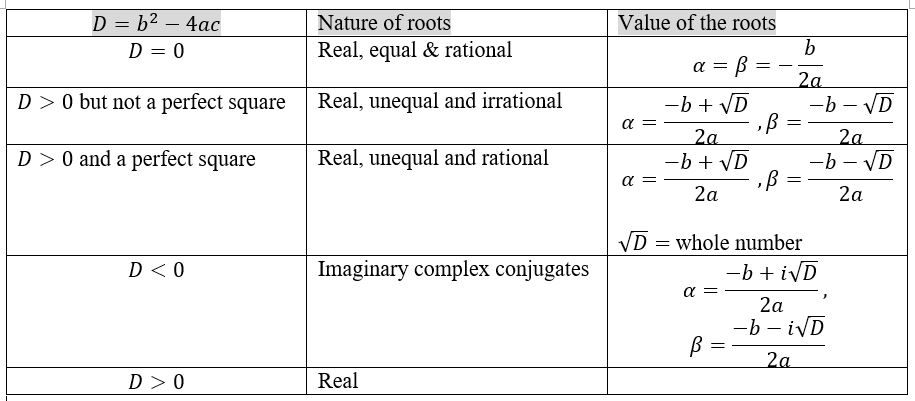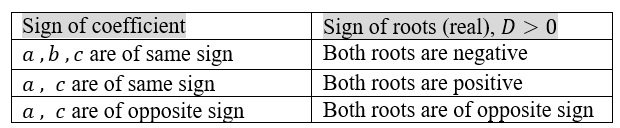Roots of the equation  $a{x}^{2}+bx+c=0$

Multiplying the equation  $a{x}^{2}+bx+c=0$   both sides by  $4a$ , we get

$4{a}^{2}{x}^{2}+4abx=–4ac$

Adding  ${b}^{2}$  to both sides

$4{a}^{2}{x}^{2}+4abx+{b}^{2}={b}^{2}–4ac$

${\left(2ax+b\right)}^{2}={b}^{2}–4ac$

$2ax+b=±\sqrt{{b}^{2}–4ac}$

Sum and Product of the roots:

If  $\alpha$  and  $\beta$  are the two roots of the above equation, then

$\alpha =\frac{–b+\sqrt{{b}^{2}–4ac}}{2a},$    $\beta =\frac{–b±\sqrt{{b}^{2}–4ac}}{2a}$

Sum of the roots =  $=\frac{–2b}{2a}=–\frac{b}{a}$

Product of the roots:

$\alpha \beta =\frac{{\left(–b\right)}^{2}–{\left[\sqrt{{b}^{2}–4ac}\right]}^{2}}{4{a}^{2}}$   $=\frac{{b}^{2}–{b}^{2}+4ac}{4{a}^{2}}=\frac{4ac}{4{a}^{2}}=\frac{c}{a}$

Nature of the roots:Transformation of equations:

Let  $\alpha$ and  $\beta$  are the roots of the equation  $a{x}^{2}+bx+c=0$

To find the equation whose roots are :

(i) Negative of the roots of the equation  $a{x}^{2}+bx+c=0$

The required roots are  $–\alpha$   and   $–\beta$ .

This can be obtained by substituting

$⇒x=–y$

so,  $a{\left(–y\right)}^{2}+b\left(–y\right)+c=0$   $⇒a{y}^{2}–by+c=0$

or       $a{x}^{2}+bx+c=0$

(ii) Increased by  $h$  i.e.

substituting  $y=\alpha +h=x+h$   $⇒x=y–h$

so,  $a{\left(y–h\right)}^{2}+b\left(y–h\right)+c=0$

$⇒a{y}^{2}+y\left(b–2ah\right)+\left(a{h}^{2}–bh+c\right)=0$

so the required equation is

$a{x}^{2}+x\left(b–2ah\right)+\left(a{h}^{2}–bh+c\right)=0$

Sign of coefficient determining the sign of both real roots of $a{x}^{2}+bx+c=0$